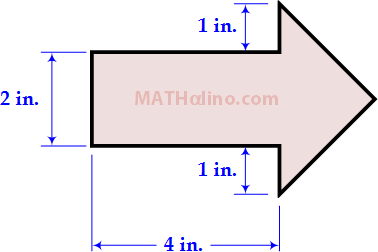# Area of Arrow Polygon

2 posts / 0 new
Jhun VertArea of Arrow Polygon

The vertex angle at the tip of the arrow is 90 degrees. Calculate the area of the arrow-shaped polygon.esmilitarThe Total Area of Arrow Polygon= Area of Rectangle + Area of Right Triangle or Isosceles Right Triangle.
Since the vertex angle at the tip of the arrow is 90 degrees by inspection the triangle is isosceles triangle in which two sides are equal, We all know that the total interior angles of a triangle is equal to 180 degrees, thus the two remaining angles are 45 degrees each.Here i divided the triangle into two equal parts from the end side midpoint of a rectangle vertically and horizontally to the vertex with two sides at 2 inches each which is also a right triangle or isosceles right triangle.
Total Area of the Arrow polygon would be=( 2 x 4) + 2 ((1/2) (2)(2))
Total Area of the Arrow Polygon= 8 + 4 = 12 sq. in Ans.

Subscribe to MATHalino on